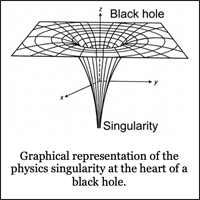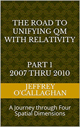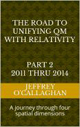Please click here if you would like to read or save to an HTML file a summary the ideas presented in this blog.

In an earlier post An alternative to a singularity? Aug 15 2008 we defined what happens to matter and energy as it falls into a black hole in terms of inertial reference frames. However, we did not attempt to define what happens after that. The reason was because we must use Einstein’s mathematical definitions of the curvature in the geodesics that define how mass and energy move in a space-time environment to do so.  They tells us that it would take an infinite amount of time mass and energy to form singularity after passing through the event horizon of a black hole for the same reason we that observe it to take an infinite amount of time to reach it from the outside.This is because as mass or energy is added to it the curvature defining its gravitational geodesic in space time expands adding another layer to it. However that does not mean that the matter or energy that is under that layer is free to move towards its center because the gravitational curvature in the geodesic that defines it movement is still there but at a lower gravitational potential. This means any matter or energy that exits at a layer under the event horizon could NOT move towards its center to form a singularity but can only move around the circular geodesic generated by the gravitational potential at that level. However, Einstein’s math tells us would take infinite amount of time to cross to a lower gravitational level. This is similar to the observations involving how matter and energy that tell us it take an infinite amount of time for it move through an event horizon from outside of black hole.

This tells us that either we have misinterpreted the math that tells us that a singular can exist at the center of a black hole or we must rewrite them based on the observations of how mass and energy interact with the event horizon of a black hole. We do not believe we have any other options base on those observations.

So if a singularly is not at the center of a black hole what is.

We know the densest form of observable matter is found in a neutron star where the gravitational forces are strong enough to overcome the forces keeping electrons protons and neutron apart. We also know that a neutron star is capable of becoming a black hole if it absorbs enough mass and energy to become a one. However, that does not mean that it collapses to a singularity. The total energy and therefore the total gravitational potential of the volume of space our solar system occupies consists of the mass and energy of the sun and the planets which are orbiting it. This observation suggests that the total mass in the volume of space occupied by a black hole maybe made up of the components of neutron star and the mass that as was show above would be orbiting it on the gravitational geodesic created by it. These observations of our solar system suggest that the central core of a black hole is NOT a singularity but the remnants of neutron star whose gravitational potential has been increase enough by the mass and energy that is orbiting in the gravitational geodesic created by it to form one.

 The Road to Unifying QM with Relativity part 1 2007 thru 2010Ebook \$8.00Paper Back \$15.00 The Road to Unifying QM with Relativity part 2 2011 thru 2014Ebook \$8.00Paper Back \$16.00 The Road to Unifying QM with Relativity part 3 2015 thru 2020Ebook \$8.00Paper Back \$18.00